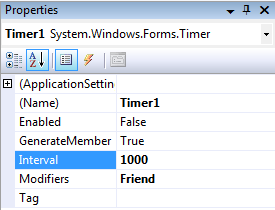# How to Make a Stopwatch in vb.net using Timer

Tip Abstraction:
This tutorial says that how to create a simple stopwatch using Visual Studio vb.net Programming.

Creating a stopwatch is interesting.  In Visual Basic, Timer Control helps you to create a stopwatch.
Mainly there are two methods to create a stopwatch. First method is simple method which uses a number and increments each timer ticks. Second method catches elapsed time of timer and declares as a time span.
Here we use first simple method to create our stopwatch.

First of all add three labels and give name as ‘00’ for each. And add a ‘:’ label between them.
Here first, second and third labels named with ‘hourtxt’, ‘minutetxt’ and ‘secondtxt’ respectively.Now add three buttons to the form and give name as Start/Resume, pause and reset as shown in figure.
Now add a timer to the form. Just drag down from Toolbox (Under components section)Now go to the properties of the Timer1. (Right click on timer1 and take Properties). Then set Interval into 1000.Now we are going to the write VB codes. First of all open code section and at starting section declare three common variables to keep the values of second, minute and hour.

`Public Class Form1`

Dim second As Integer = 0

Dim minute As Integer = 0

Dim Hour As Integer = 0

End Class

Timer is a tool which runs always. We already set an interval for the timer1. 1000 milliseconds mean 1 second.
Just double click on the timer1 icon; here you have to write our major codes for the stopwatch.
You will get an event of timer1_tick like this

`Private Sub Timer1_Tick(ByVal sender As System.Object, ByVal e As System.EventArgs) Handles Timer1.Tick`

End Sub

You need to write code inside these lines:

`        second = second + 1`

secondtxt.Text = second

If (second = 60) Then

minute = minute + 1

second = 0

minutetxt.Text = minute

End If

If (minute = 60) Then

Hour = Hour + 1

second = 0

minute = 0

minutetxt.Text = minute

hourtxt.Text = Hour

End If

### Code Explanation:

second = second + 1

At every seconds of timer1 tick, the value of variable ‘second’ will incremented one by one and set its value into the label ‘secondtxt’.

`If (second = 60) Then`

minute = minute + 1

minutetxt.Text = minute

second = 0

End If

Whenever variable ‘second’ reach the value 60, then the value of minutes will be incremented by one and the value of minute will be saved into minutetxt. And the value of second will be again 0.

`        If (minute = 60) Then`

Hour = Hour + 1

hourtxt.Text = Hour

second = 0

minute = 0

minutetxt.Text = minute

End If

Like the logic of minutes, whenever minutes reach the value 60, then the value of ‘hour’ will be incremented by one.

When we click on the button Start/Resume:

```Private Sub Button12_Click(ByVal sender As System.Object, ByVal e As System.EventArgs) Handles Button12.Click

Timer1.Start()

End Sub```

Then timer1 will be started to run.

While we press on pause button:

` Private Sub Button14_Click(ByVal sender As System.Object, ByVal e As System.EventArgs) Handles Button14.Click`

Timer1.Stop()

End Sub

Then the timer1 will be stopped its run.

When timer get reset:

`Private Sub Button13_Click(ByVal sender As System.Object, ByVal e As System.EventArgs) Handles Button13.Click`

Timer1.Stop()

minute = 0

second = 0

Hour = 0

secondtxt.Text = “00”

minutetxt.Text = “00”

hourtxt.Text = “00”

End Sub

Then everything will be reset by assigning values as 0 and texts into ‘00’.

### The complete code:

`Public Class Form1`

Dim second As Integer = 58

Dim minute As Integer = 10

Dim Hour As Integer = 0

Private Sub Timer1_Tick(ByVal sender As System.Object, ByVal e As System.EventArgs)    Handles Timer1.Tick

second = second + 1

secondtxt.Text = second

If (second = 60) Then

minute = minute + 1

second = 0

minutetxt.Text = minute

End If

If (minute = 60) Then

Hour = Hour + 1

second = 0

minute = 0

minutetxt.Text = minute

hourtxt.Text = Hour

End If
End Sub

Private Sub Button15_Click(ByVal sender As System.Object, ByVal e As System.EventArgs)

Timer1.Start()

End Sub

Private Sub Button14_Click(ByVal sender As System.Object, ByVal e As System.EventArgs) Handles Button14.Click

Timer1.Stop()

End Sub
Private Sub Button13_Click(ByVal sender As System.Object, ByVal e As System.EventArgs) Handles Button13.Click

Timer1.Stop()

minute = 0

second = 0

Hour = 0

secondtxt.Text = “00”

minutetxt.Text = “00”

hourtxt.Text = “00”

End Sub

End Class

### Sample Output: